Next: Inferring in absence of Up: Inferring the success parameter Previous: Introduction

# The binomial distribution and its inverse problem

An important class of counting experiments can be modeled as independent Bernoulli trials. In each trial we believe that a success will occur with probability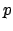, and a failure with probability. If we considerindependent trials, all with the same probability, we might be interested in the total number of successes, independently of their order. The total number of successescan range between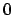and, and our belief on the outcome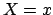can be evaluated from the probability of each success and some combinatorics. The result is the well known binomial distribution, hereafter indicated with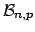:(1)

having expected value and standard deviation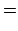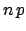(2)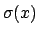(3)

We associate the formal quantities expected value and standard deviation to the concepts of (probabilistic) prevision and standard uncertainty.

The binomial distribution describes what is sometimes called a direct probability problem, i.e. calculate the probability of the experimental outcome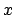(the effect) givenand an assumed value of. The inverse problem is what concerns mostly scientists: infergivenand. In probabilistic terms, we are interested in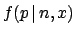. Probability inversions are performed, within probability theory, using Bayes theorem, that in this case reads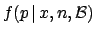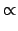(4)

where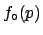is the prior,the posterior (or final) and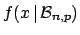the likelihood. The proportionality factor is calculated from normalization. [Note the use of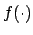for the several probability functions as well as probability density functions (pdf), also within the same formula.] The solution of Eq. (4), related to the names of Bayes and Laplace, is presently a kind of first text book exercise in the so called Bayesian inference (see e.g. Ref. [2,3]). The issue of priors in this kind of problems will be discussed in detail in Sec. 3.1, especially for the critical cases ofand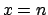.

The problem can be complicated by the presence of background. This is the main subject of this paper, and we shall focus on two kinds of background.

a)
Background can only affect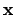. Think, for example, of a person shootingtimes on a target, and counting, at the end, the numbers of scoresin order to evaluate his efficiency. If somebody else fires by mistake at random on his target, the numberwill be affected by background. The same situation can happen in measuring efficiencies in those situations (for example due to high rate or loose timing) in which the time correlation between the equivalents of shooting' and scoring' cannot be done on a event by event basis (think, for example, to neutron or photon detectors).

The problem will be solved assuming that the background is described by a Poisson process of well known intensity, that corresponds to a well known expected valueof the resulting Poisson distribution (in the time domain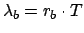, whereis measuring time). In other words, the observedis the sum of two contributions: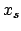due to the signal, binomially distributed with, plus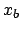due to background, Poisson distributed with parameter, indicated by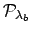.

For large numbers (and still relatively low background) the problem is easy to solve: we subtract the expected number of background and calculate the proportion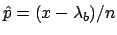. For small numbers, the estimator'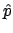can become smaller than 0 or larger then 1. And, even ifcomes out in the correct range, it is still affected by large uncertainty. Therefore we have to go through a rigorous probability inversion, that in this case is given by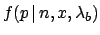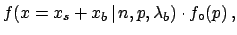(5)

where we have written explicitly in the likelihood thatis due to the sum of two (individually unobservable!) contributionsand(hereafter the subscriptsandstand for signal and background.)

b)
The background can show up, at random, as independent fake' trials, all with the sameof producing successes. An example, that has indeed prompted this paper, is that of the measuring the proportion of blue galaxies in a small region of sky where there are galaxies belonging to a cluster, as well as background galaxies, the average proportion of blue galaxies of which is well known. In this case bothandhave two contributions: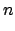(6)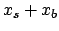(7)

with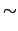(8)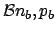(9)(10)

where' stands for follows a given distribution'.

Again, the trivial large number (and not too large background) solution is the proportion of background subtracted numbers,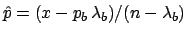. But in the most general case we need to inferfrom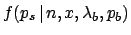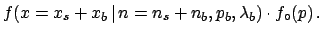(11)

We might be also interested also to other questions, like e.g. how many of theobject are due to the signal, i.e.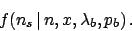Indeed, the general problem lies in the joint inferencefrom which we can get other information, like the conditional distribution of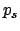for any given number of events attributed to signal: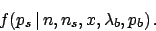Finally, we may also be interested in the rate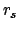of the signal objects, responsible of thesignal objects in the sample (or, equivalently, to the Poisson distribution parameter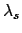):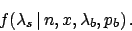Next: Inferring in absence of Up: Inferring the success parameter Previous: Introduction
Giulio D'Agostini 2004-12-13